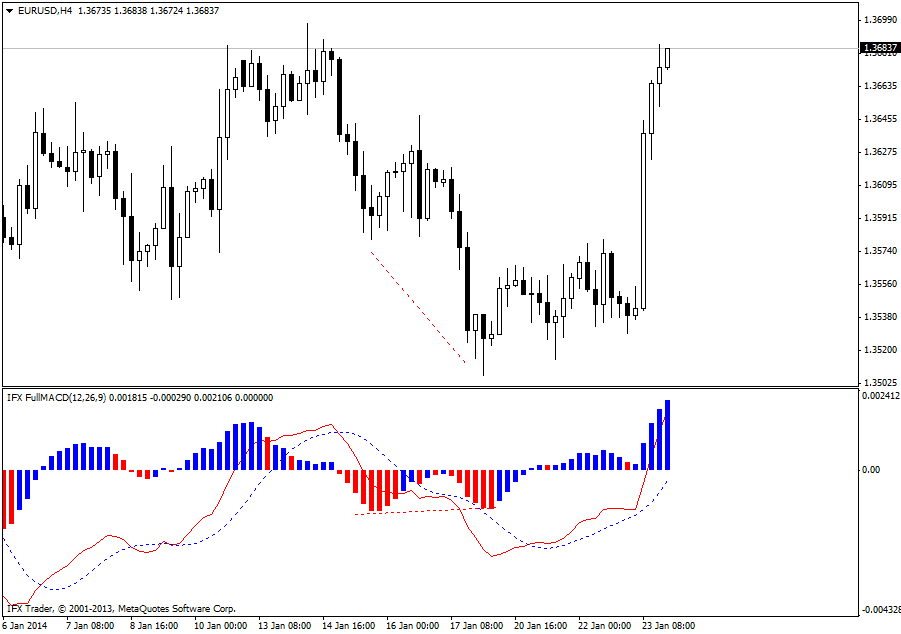#### 公式

MACD = (ЕМАs(P) - EMAl(P)),

Signal = SМАa(ЕМАs(P) - EMAl(P)), where

ЕМАl(P) is a long-term exponential moving average;

EMAs(P) is a short-term exponential moving average;

SМАa(P) is a short-term simple moving average;

P is a closing price.

#### 交易应用

MACD是一种经典的市场震荡指标，在趋势市场和横盘市场中均可提供清晰的信号。相较只能在市场整理期间应用的随机震荡指标，MACD的功能更加丰富。#### InstaForex 完整MACD参数

Fast_MA_Period = 12

Slow_MA_Period = 26

Signal_MA_Period = 9